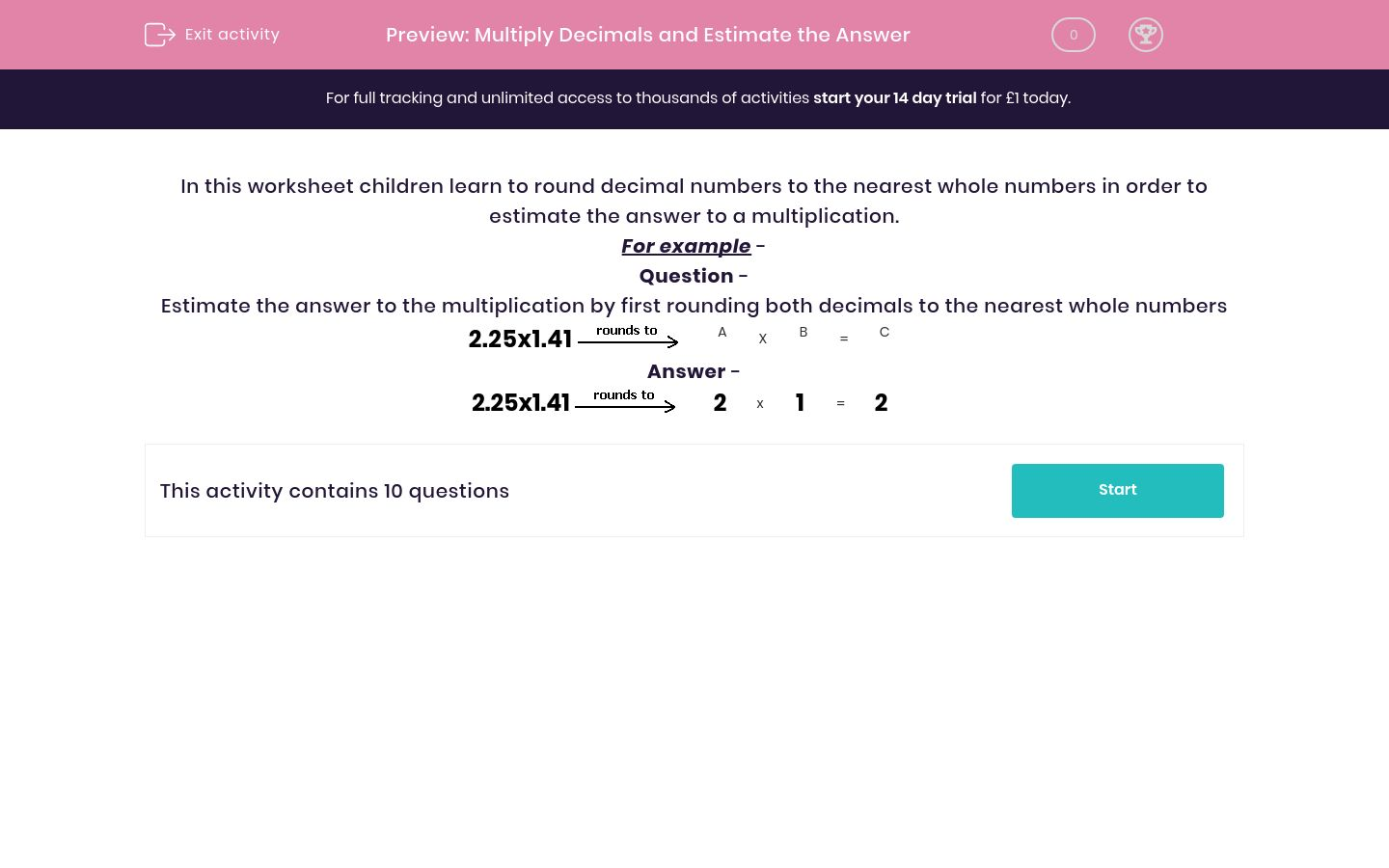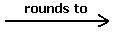# Multiply Decimals and Estimate the Answer

In this worksheet, students round decimals to the nearest whole numbers and then estimate the answer to a multiplication.Key stage:  KS 2

Curriculum topic:   Maths and Numerical Reasoning

Curriculum subtopic:   Decimals

Difficulty level:### QUESTION 1 of 10

In this worksheet children learn to round decimal numbers to the nearest whole numbers in order to estimate the answer to a multiplication.

For example -

Question -

Estimate the answer to the multiplication by first rounding both decimals to the nearest whole numbers

2.25x1.41A
X
 B
=
 C

2.25x1.412
x
 1
=
 2

Estimate the answer to the multiplication by first rounding both decimals to the nearest whole numbers.

7.43X5.1A
X
 B
=
 C

Remember to put your answers in the same sequence as the question.

For example, will be 7.43 to the nearest whole number and B will be 5.1...

## Column B

A
35
B
5
C
7

Estimate the answer to the multiplication by first rounding both decimals to the nearest whole numbers.

8.45X6.28A
X
 B
=
 C

Remember to put your answers in the same sequence as the question.

For example, will be 8.45 to the nearest whole number and B will be 6.28...

## Column B

A
48
B
8
C
6

Estimate the answer to the multiplication by first rounding both decimals to the nearest whole numbers.

9.93X3.19A
X
 B
=
 C

Remember to put your answers in the same sequence as the question.

For example, will be 9.93 to the nearest whole number and B will be 3.19...

## Column B

A
30
B
3
C
10

Estimate the answer to the multiplication by first rounding both decimals to the nearest whole numbers..

5.83X2.75A
X
 B
=
 C

Remember to put your answers in the same sequence as the question.

For example, will be 5.83 to the nearest whole number and B will be 2.75...

## Column B

A
18
B
3
C
6

Estimate the answer to the multiplication by first rounding both decimals to the nearest whole numbers.

7.15X1.2A
X
 B
=
 C

Remember to put your answers in the same sequence as the question.

For example, will be 7.15 to the nearest whole number and B will be 1.2...

## Column B

A
7
B
7
C
1

Estimate the answer to the multiplication by first rounding both decimals to the nearest whole numbers.

3.27X1.12A
X
 B
=
 C

Remember to put your answers in the same sequence as the question.

For example, will be 3.27 to the nearest whole number and B will be 1.12...

## Column B

A
3
B
3
C
1

Estimate the answer to the multiplication by first rounding both decimals to the nearest whole numbers.

9.08X2.47A
X
 B
=
 C

Remember to put your answers in the same sequence as the question.

For example, will be 9.08 to the nearest whole number and B will be 2.47...

## Column B

A
9
B
2
C
18

Estimate the answer to the multiplication by first rounding both decimals to the nearest whole numbers.

1.8X1.71A
X
 B
=
 C

Remember to put your answers in the same sequence as the question.

For example, will be 1.8 to the nearest whole number and B will be 1.71...

## Column B

A
2
B
2
C
4

Estimate the answer to the multiplication by first rounding both decimals to the nearest whole numbers.

5.33X4.68A
X
 B
=
 C

Remember to put your answers in the same sequence as the question.

For example, will be 5.33 to the nearest whole number and B will be 4.68...

## Column B

A
25
B
5
C
5

Estimate the answer to the multiplication by first rounding both decimals to the nearest whole numbers.

7.17X2.3A
X
 B
=
 C

Remember to put your answers in the same sequence as the question.

For example, will be 7.17 to the nearest whole number and B will be 2.3...

## Column B

A
14
B
2
C
7
• Question 1

Estimate the answer to the multiplication by first rounding both decimals to the nearest whole numbers.

7.43X5.1A
X
 B
=
 C

Remember to put your answers in the same sequence as the question.

For example, will be 7.43 to the nearest whole number and B will be 5.1...

## Column B

A
7
B
5
C
35
• Question 2

Estimate the answer to the multiplication by first rounding both decimals to the nearest whole numbers.

8.45X6.28A
X
 B
=
 C

Remember to put your answers in the same sequence as the question.

For example, will be 8.45 to the nearest whole number and B will be 6.28...

## Column B

A
8
B
6
C
48
• Question 3

Estimate the answer to the multiplication by first rounding both decimals to the nearest whole numbers.

9.93X3.19A
X
 B
=
 C

Remember to put your answers in the same sequence as the question.

For example, will be 9.93 to the nearest whole number and B will be 3.19...

## Column B

A
10
B
3
C
30
• Question 4

Estimate the answer to the multiplication by first rounding both decimals to the nearest whole numbers..

5.83X2.75A
X
 B
=
 C

Remember to put your answers in the same sequence as the question.

For example, will be 5.83 to the nearest whole number and B will be 2.75...

## Column B

A
6
B
3
C
18
• Question 5

Estimate the answer to the multiplication by first rounding both decimals to the nearest whole numbers.

7.15X1.2A
X
 B
=
 C

Remember to put your answers in the same sequence as the question.

For example, will be 7.15 to the nearest whole number and B will be 1.2...

## Column B

A
7
B
1
C
7
• Question 6

Estimate the answer to the multiplication by first rounding both decimals to the nearest whole numbers.

3.27X1.12A
X
 B
=
 C

Remember to put your answers in the same sequence as the question.

For example, will be 3.27 to the nearest whole number and B will be 1.12...

## Column B

A
3
B
1
C
3
• Question 7

Estimate the answer to the multiplication by first rounding both decimals to the nearest whole numbers.

9.08X2.47A
X
 B
=
 C

Remember to put your answers in the same sequence as the question.

For example, will be 9.08 to the nearest whole number and B will be 2.47...

## Column B

A
9
B
2
C
18
• Question 8

Estimate the answer to the multiplication by first rounding both decimals to the nearest whole numbers.

1.8X1.71A
X
 B
=
 C

Remember to put your answers in the same sequence as the question.

For example, will be 1.8 to the nearest whole number and B will be 1.71...

## Column B

A
2
B
2
C
4
• Question 9

Estimate the answer to the multiplication by first rounding both decimals to the nearest whole numbers.

5.33X4.68A
X
 B
=
 C

Remember to put your answers in the same sequence as the question.

For example, will be 5.33 to the nearest whole number and B will be 4.68...

## Column B

A
5
B
5
C
25
• Question 10

Estimate the answer to the multiplication by first rounding both decimals to the nearest whole numbers.

7.17X2.3A
X
 B
=
 C

Remember to put your answers in the same sequence as the question.

For example, will be 7.17 to the nearest whole number and B will be 2.3...

## Column B

A
7
B
2
C
14
---- OR ----

Sign up for a £1 trial so you can track and measure your child's progress on this activity.

### What is EdPlace?

We're your National Curriculum aligned online education content provider helping each child succeed in English, maths and science from year 1 to GCSE. With an EdPlace account you’ll be able to track and measure progress, helping each child achieve their best. We build confidence and attainment by personalising each child’s learning at a level that suits them.

Get started